# ePSproc Matlab demo

As noted in the main readme/intro doc, ePSproc originally started as a set of Matlab routines, before migration to Python in 2019. Although no longer maintained/updated since ~2018, the Matlab routines are still available as part of the ePSproc distribution. This notebook demos the routines, following the demo script ePSproc_NO2_MFPADs_demo.m (to run this code in a Jupyter environment, a Matlab kernel is required, e.g. Calysto’s Matlab kernel).

Functionality:

• Read raw photoionization matrix elements from ePS output files with “dumpIdy” segments

• Plot X-sects

• (Beta testing): Calculate MF-BLMs from matrix elements, see ePSproc_MFBLM.m

• (Under development): Calculate AF-BLMs from matrix elements.

Source:

• /matlab: stable matlab code (as per release v1.0.1 <https://github.com/phockett/ePSproc/releases>__).

• a set of functions for processing (ePSproc*.m files)

• a script showing demo calculations, ePSproc_NO2_MFPADs_demo.m

• the benchmark results from these calculations, ePSproc_NO2_testing_summary_250915.pdf

• additional notes on ePS photoionization matrix elements, ePSproc_scattering_theory_ePS_notes_011015.pdf.

See ePSproc: Post-processing suite for ePolyScat electron-molecule scattering calculations <https://www.authorea.com/users/71114/articles/122402/_show_article>_ for more details.

## Setup

:

cd('~/github/ePSproc')  % Change to ePSproc root dir.


ans =

'9.4.0.813654 (R2018a)'


:

%% *** SETTINGS
%  Set up basic environment

% Name & path to ePS output file. In this version set full file name here, and working directory below.
% fileName='no2_demo_ePS.out'  % OK for MFPAD testing, but only single E point
fileName='n2_3sg_0.1-50.1eV_A2.inp.out'

% Set paths for Linux or Win boxes (optional!)
if isunix
dirSlash='/';
else
dirSlash='\';
end

filePath=[pwd dirSlash 'data' dirSlash 'photoionization'];                       % Root to working directory, here set as current dir/data/photoionization
fileBase=[filePath dirSlash fileName];   % Full path to ePS results file, here set as current working direcory

scriptPath=[pwd dirSlash 'matlab' dirSlash];  % Add path to ePSproc scrips to Matlab path list, here set as current dir/matlab
path(path,[scriptPath]);


fileName =

'n2_3sg_0.1-50.1eV_A2.inp.out'


:

%% *** Read data
%  Variables:
%       rlAll contains matrix elements (from DumpIdy segments)
%       params contains various calculation parameters
%       getCro contains cross-section (from GetCro segments), if present

params.fileBase=fileBase;
params.fileName=fileName;

*** Reading ePS output file
/home/paul/github/ePSproc/data/photoionization/n2_3sg_0.1-50.1eV_A2.inp.out
Found 102 sets of matrix elements
Read 102 sets of matrix elements (0 blank records)
Found 2 symmetries
'SU'    'PU'

Found 51 energies
Found 2 atoms
Found 18 data records
Found 3 sets of cross sections

:

% Matrix elements are stored in a structure
rlAll


rlAll =

2x51 struct array with fields:

eKE
PE
symm
symmSet
MbNorm
rawIdy1
rlnl1
rawIdy2
rlnl2
pWaveAll


:

% GetCro outputs (total cross-secions, LF betas)
getCro


getCro =

1x3 struct array with fields:

GetCro


:

% General calculation params
params


params =

struct with fields:

symmList: {'SU'  'PU'  'All'}
eKE: [1x51 double]
symmAll: {1x102 cell}
eAll: [1x102 double]
nRecords: 102
nEnergies: 51
nSymms: 2
gLmax: 11
blankRec: 0
missingE: [1x0 double]
pWaveAll: [144x51x3 double]
pWaveAllMb: [144x51x3 double]
LMallInd: [144x2 double]
coords: {1x5 cell}
dataRecords: {18x2 cell}
IP: 15.5800
fileBase: '/home/paul/github/ePSproc/data/photoionization/n2_3sg_0.1-50.1eV_A2.inp.out'
fileName: 'n2_3sg_0.1-50.1eV_A2.inp.out'



## Plot cross-sections and betas

These are taken from the GetCro segments in the ePS output files, and correspond to results for an isotropic ensemble of molecules, i.e. observables in the lab frame (LF) for 1-photon ionization (see the ePS tutorial for more details).

:

%% Plot GetCro results for each symm & total

col=2;  % Select column from getCro output (see params.GetCroHeader)

figure('color',[1 1 1],'name','GetCro outputs');

for n=1:length(getCro)
plot(getCro(n).GetCro(:,1)-params.IP,getCro(n).GetCro(:,col));
hold on;
end

title({['NO_2 ePS resutls, files ' strrep(fileName,'_','\_')]; 'X-sects from ePS(GetCro) results'});
xlabel('eKE/eV');
ylabel('X-sect/Mb');

legend([params.symmList 'Sum']);

Warning: MATLAB has disabled some advanced graphics rendering features by switching to software OpenGL. For more information, click <a href="matlab:opengl('problems')">here</a>.
Warning: Ignoring extra legend entries.
> In legend>set_children_and_strings (line 646)
In legend>make_legend (line 316)
In legend (line 259)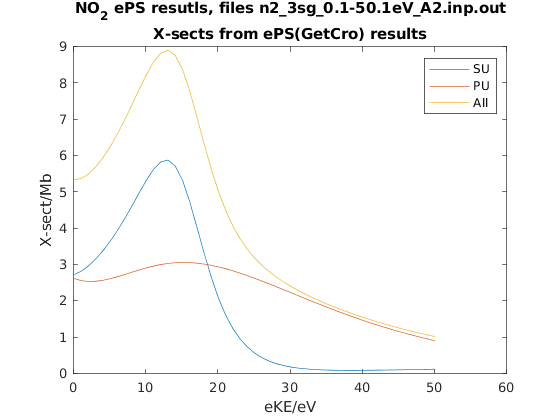These are calculated numerically from the matrix elements, for a given polarization geometry and symmetry (the method is the same as the python version of the routine).

:

%% *** Calculate MFPADs - single polarization geometry, all energies and symmetries
%  Calculate for specified Euler angles (polarization geometry) & energies

% Set resolution for calculated I(theta,phi) surfaces
res=100;

% ip components to use from ePS output (1=length gauge, 2=velocity gauge)
ipComponents=1;

% it components to use from ePS output (for degenerate cases), set an array here for as many components as required, e.g. it=1, it=[1 2] etc.
it=1;

% Set light polarization and axis rotations LF -> MF
p=0;                % p=0 for linearly pol. light, +/-1 for L/R circ. pol.
eAngs=[0 0 0];      % Eugler angles for rotation of LF->MF, set as [0 0 0] for z-pol, [0 pi/2 0] for x-pol, [pi/2 pi/2 0] for y-pol
polLabel='z';

% Run calculation - outputs are D, full set of MFPADs (summed over symmetries); Xsect, calculated X-sects; calcsAll, structure with results for all symmetries.

% Add pol labels - currently expected in plotting routine, but not set in MFPAD routine
for n=1:size(calcsAll,2)
for symmIn=1:size(calcsAll,1)
calcsAll(symmInd,n).polLabel=polLabel;
end
end

:

% Results are output as a structure, dims (symmetries, energies).
calcsAll


calcsAll =

3x51 struct array with fields:

D
C
Cthres
eKE
symm
euler
Xsect
XsectD
Rlf
p
Cind


:

%plot -s 800,400


(Above is a line-magic for setting displayed plot size with the Calysto kernel - see the demo notebook for more.)

:

%% Plotting - MFPAD panel plots

% Set plot ranges
symmInd=1;     % Select symmetry (by index into calcsAll rows). Final symmetry state is set as sum over all symmetries
% eRange=1;      % Select energies (by index into calcsAll cols)
eRange=1:3:20;

sPlotSet=[2 4];             % Set [rows cols] for subplot panels. The final panel will be replaced with a diagram of the geometry
% titlePrefix='NO2 testing';  % Set a title prefix for the figure
titlePrefix='';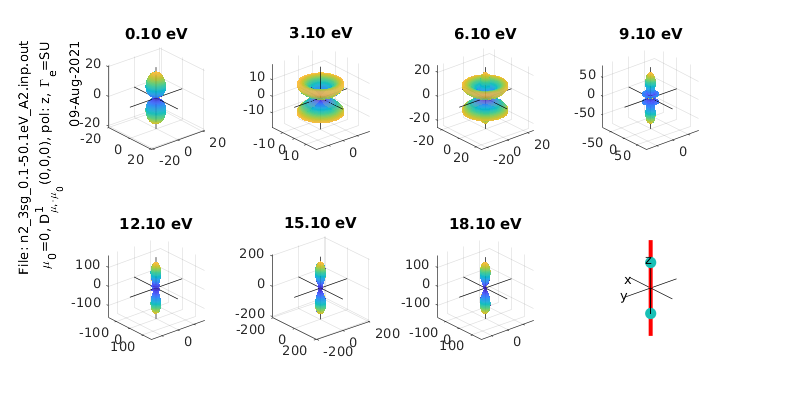:

% Calculate & plot for a different polarization state
eAngs = [0 pi/2 0];  % x-pol case
polLabel = 'x';

% Add pol labels - currently expected in plotting routine, but not set in MFPAD routine
for n=1:size(calcsAll,2)
for symmIn=1:size(calcsAll,1)
calcsAll(symmInd,n).polLabel=polLabel;
end
end

symmInd=3;


symmInd =

3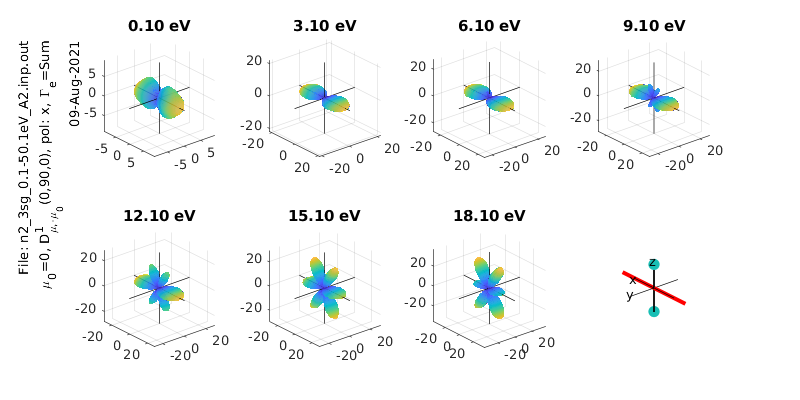:

% Calculate & plot for a different polarization state
eAngs = [0 pi/4 0];  % Diagonal pol case
polLabel = 'x';

% Add pol labels - currently expected in plotting routine, but not set in MFPAD routine
for n=1:size(calcsAll,2)
for symmIn=1:size(calcsAll,1)
calcsAll(symmInd,n).polLabel=polLabel;
end
end

% Plot all symmetries
for symmInd = 1:3

end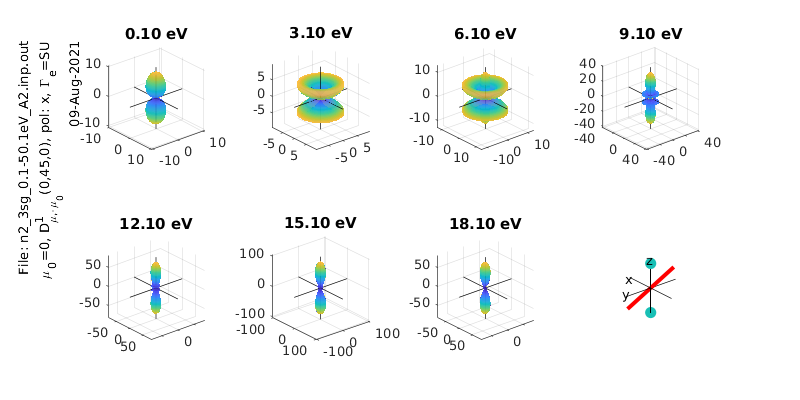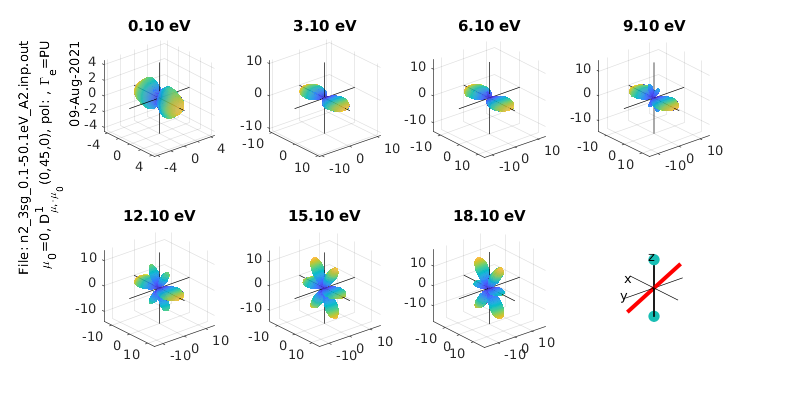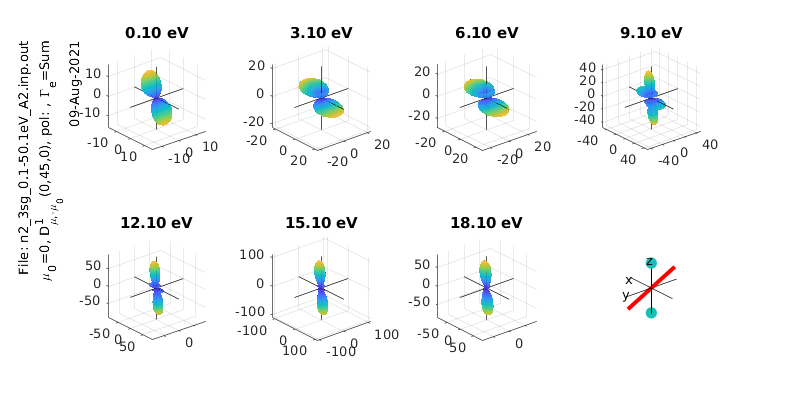## MF $$\beta_{LM}$$

:

%% *** Calculate MFPADs - single polarization geometry, all energies and symmetries
%  Calculate for specified Euler angles (polarization geometry) & energies

% Set resolution for calculated I(theta,phi) surfaces
res=100;

% ip components to use from ePS output (1=length gauge, 2=velocity gauge)
ipComponents=1;

% it components to use from ePS output (for degenerate cases), set an array here for as many components as required, e.g. it=1, it=[1 2] etc.
it=1;

% Set light polarization and axis rotations LF -> MF
p=0;                % p=0 for linearly pol. light, +/-1 for L/R circ. pol.
eAngs=[0 0 0];      % Eugler angles for rotation of LF->MF, set as [0 0 0] for z-pol, [0 pi/2 0] for x-pol, [pi/2 pi/2 0] for y-pol
polLabel='z';

% Run calculation - outputs are D, full set of MFPADs (summed over symmetries); Xsect, calculated X-sects; calcsAll, structure with results for all symmetries.

% Add pol labels - currently expected in plotting routine, but not set in MFPAD routine
% for n=1:size(calcsAll,2)
%     for symmIn=1:size(calcsAll,1)
%         calcsAll(symmInd,n).polLabel=polLabel;
%     end
% end

:

plot(calcsAll.')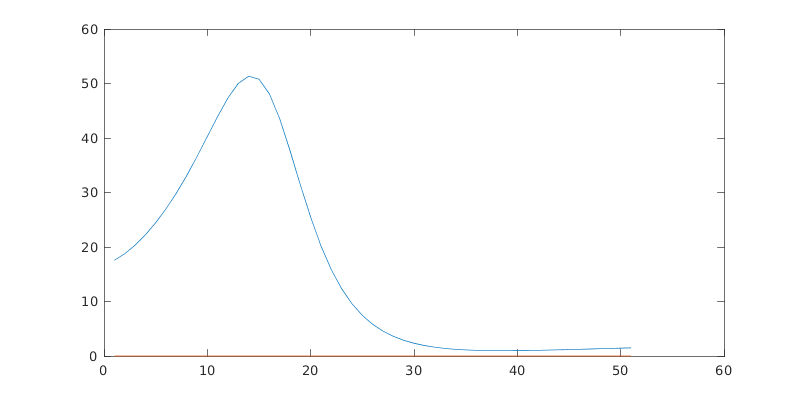[ ]: